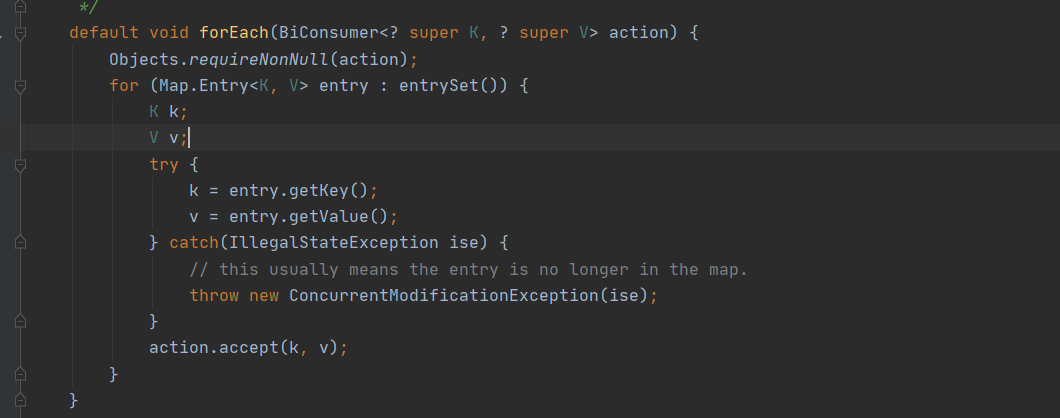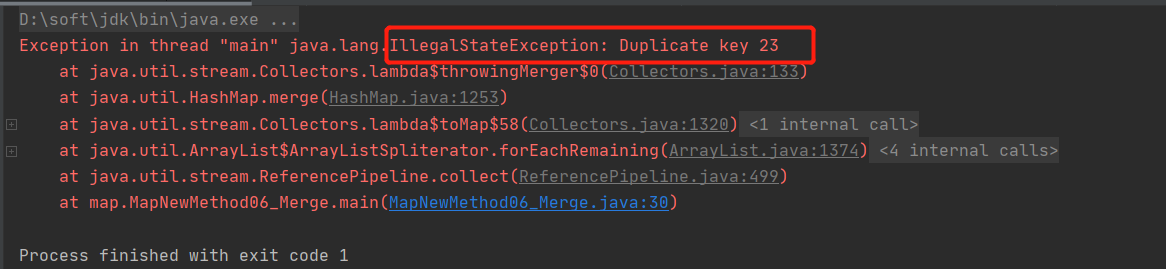## 【JAVA8】Map新方法，别再重复造车轮了_保护我方胖虎的博客-程序员宝宝_java8 map新方法

### 文章目录

java8 发布后，map类库增加了多个新的方法，利用这些新方法能更加便利地操纵Map中的数据

``````getOrDefault，forEach，compute，computeIfAbsent，computeIfPresent，merge，putIfAbsent，remove(key,value)，replace，replaceAll
``````

# getOrDefault

getOrDefault() 方法获取指定 key 对应对 value，如果找不到 key ，则返回设置的默认值。

``````hashmap.get(Object key, V defaultValue)
``````

• key - 键
• defaultValue - 当指定的key并不存在映射关系中，则返回的该默认值

``````Map<String, Integer> map = new LinkedHashMap<>(4);
map.put("lisa", 1);
map.put("jone", 2);
map.put("selina", 3);
``````

java8之前的方式

``````Map<String, Integer> map = new HashMap<>(4);
map.put("lisa", 1);
int lisaValue = 0;
if (map.containsKey("lisa")) {

lisaValue = map.get("lisa");
}
System.out.println(lisaValue);
``````

java8

``````// key为 lisa 的映射存在则返回对应value,否则返回默认值-1
Integer java8lisaValue = map.getOrDefault("lisa", -1);
// 1
System.out.println(java8lisaValue);
``````

# forEach

foreach 可以让我们以Lambda的方式快速遍历映射集（map）

``````map.forEach(BiConsumer<K, V> action)
``````

• `BiConsumer`- java8新提供的函数式接口，我们可以定义遍历消费逻辑

``````Map<String, Integer> map = new LinkedHashMap<>(4);
map.put("lisa", 1);
map.put("jone", 2);
map.put("selina", 3);
``````

java8之前的方式

``````for (Map.Entry<String, Integer> entry : map.entrySet()) {

System.out.println(entry.getKey() + "==" + entry.getValue());
}
``````

java8

`````` map.forEach((k, v) -> System.out.println(k + "=" + v));
``````# compute

``````map.compute(K key, BiFunction remappingFunction)
``````

• key - 键
• remappingFunction - 重新映射函数，用于重新计算值

``````Map<String, Integer> map = new LinkedHashMap<>(4);
map.put("lisa", 1);
map.put("jone", 2);
map.put("selina", 3);
``````

java8 之前操作

``````private static Integer computeBefore(String key, Integer newValue) {

Map<String, Integer> map = new LinkedHashMap<>(4);
map.put("lisa", 1);
map.put("jone", 2);
map.put("selina", 3);
Integer oldValue = map.get(key);
if (newValue != null) {

if (map.containsKey(key) && oldValue != null) {

map.remove(key);
}
return null;
} else {

map.put(key, newValue);
return newValue;
}
}
``````

java8操作

Key在Map中存在

``````// jone key存在,且 新value不为null,则会返回最新jone对应的value (v + 234),并添加到映射集map中
Integer joneNewValue = map.compute("jone", (k, v) -> v == null ? 0 : v + 234);
// joneNewValue:236
System.out.println("joneNewValue:" + joneNewValue);
// {lisa=1, jone=236, selina=3}
System.out.println(map);

System.out.println("================");
// jone key存在,且 新value为null,则会返回最新jone对应的value null,并将映射在原集中删除
Integer joneNewValue2 = map.compute("jone", (k, v) -> null);
// joneNewValue2:null
System.out.println("joneNewValue2:" + joneNewValue2);
// 此时会 移除 KEY  jone
// {lisa=1, selina=3}
System.out.println(map);
``````

Key在Map中不存在

``````// asd key不存在,且 新value不为null,则会返回最新asd对应的value 111,并保存到映射集map中
Integer asdNewValue = map.compute("asd", (k, v) -> 111);
// asdNewValue:111
System.out.println("asdNewValue:" + asdNewValue);
// {lisa=1, selina=3, asd=111}
System.out.println(map);

System.out.println("================");
// box key不存在,且 新value为null,则会返回null, 不保存到映射集map中
Integer boxNewValue = map.compute("box", (k, v) -> null);
// boxNewValue:null
System.out.println("boxNewValue:" + boxNewValue);
// {lisa=1, selina=3, asd=111}
System.out.println(map);
``````

# computeIfAbsent

``````map.computeIfAbsent(K key, Function remappingFunction)
``````

• key - 键
• remappingFunction - 重新映射函数，用于重新计算值

``````Map<String, Integer> map = new LinkedHashMap<>(4);
map.put("lisa", 1);
map.put("jone", 2);
map.put("selina", 3);
map.put("ddd", null);
``````

java8 之前操作

``````Integer lisaValue = map.get("adas");

}
``````

java8操作

``````// 原映射集无 key为 adas的键值对，且 新key  adas 对应的 value 不为null (2345) 则会将其添加到map映射集中并返回该值
// 2345
// {lisa=1, jone=2, selina=3, ddd=null, adas=2345}
System.out.println(map);

System.out.println("--------------");

// 原映射集无 key为 dml的键值对，且 新key  ml 对应的 value 为null,则忽略添加，并返回null
Integer dmlValue = map.computeIfAbsent("dml", x -> null);
// null
System.out.println(dmlValue);
// {lisa=1, jone=2, selina=3, ddd=null, adas=2345}
System.out.println(map);

System.out.println("--------------");
// 原映射集有 Key为 ddd的键值对,但ddd对应的value 为空,如果我们再次添加有效value到ddd,则会将原键值对覆盖
Integer dddNewValue = map.computeIfAbsent("ddd", x -> 0);
// 0
System.out.println(dddNewValue);
// {lisa=1, jone=2, selina=3, ddd=0, adas=2345}
System.out.println(map);
``````

# computeIfPresent

``````map.computeIfPresent(K key, BiFunction remappingFunction)
``````

• key - 键
• remappingFunction - 重新映射函数，用于重新计算值

``````Map<String, Integer> map = new LinkedHashMap<>(4);
map.put("lisa", 1);
map.put("jone", 2);
map.put("selina", 3);
map.put("ddd", null);
``````

java8之前操作

``````if (map.get("selina") != null) {

Integer oldValue = map.get("selina");
Integer newValue = 1232312;
if (newValue != null) {

map.put("selina", newValue);
} else {

map.remove("selina");
}
}
``````

java8

``````// 原映射集中存在对应KEY  ,原Value不为null,现value不为null,则覆盖原来 键值对映射
Integer selinaNewValue = map.computeIfPresent("selina", (k, v) -> 888999);
// 888999
System.out.println(selinaNewValue);
// {lisa=1, jone=2, selina=888999, ddd=null}
System.out.println(map);

System.out.println("-------------------");
// 原映射集中存在对应KEY  ,原Value不为null,现value为null,则移除对应键值对
Integer selinaNewValue2 = map.computeIfPresent("selina", (k, v) -> null);
// null
System.out.println(selinaNewValue2);
// 移除了selina
System.out.println(map);

System.out.println("-------------------");
// 原映射集中存在对应KEY  ,原Value为null,现value 不为null,  对原映射集不做任何操作
Integer dddNewValue = map.computeIfPresent("ddd", (k, v) -> 2222222);
System.out.println(dddNewValue);
System.out.println(map);

System.out.println("-------------------");
// 原映射集中不存在对应KEY  ,现value 不为null  对原映射集不做任何操作
Integer mqNewValue = map.computeIfPresent("mq", (k, v) -> -1);
System.out.println(mqNewValue);
System.out.println(map);
``````

# merge

merge() 方法会先判断指定的 key 是否存在，如果不存在，则添加键值对到 hashMap 中，如果存在则根据逻辑重新映射该KEY键值对（如何合并相同KEY的新旧VALUE）

``````map.merge(key, value, remappingFunction)
``````

• key - 键
• value - 值
• remappingFunction - 重新映射函数，用于重新计算值

``````Map<String, Integer> map = new LinkedHashMap<>(4);
map.put("lisa", 1);
map.put("jone", 2);
map.put("selina", 3);
map.put("ddd", null);
``````

``````Map<String, Integer> map = new LinkedHashMap<>(4);
map.put("lisa", 1);
map.put("jone", 2);
map.put("selina", 3);
// {lisa=1, jone=2, selina=3}
System.out.println(map);
map.put("lisa", 2);
// {lisa=2, jone=2, selina=3}
System.out.println(map);
``````

merge，可以让我们根据自己选择如何处理相同KEY的值，类似于冲突合并（怎么合并，自己决定）

``````// 采用旧值
map.merge("lisa", 100, (oldValue, newValue) -> oldValue);

// 采用新值
map.merge("lisa", 100, (oldValue, newValue) -> newValue);

// 新旧值逻辑处理合并
map.merge("lisa", 100, (oldValue, newValue) -> oldValue + newValue);
map.merge("lisa", 100, Integer::sum);
``````

java8 Stream Collectors.toMap() 方法便要我们去合并冲突，否则如果出现相同KEY的数据，或者Value为null，程序会报错！

``````User adas = new User("adas", 23);
User mlg = new User("mlg", 34);
ArrayList<User> list = new ArrayList<>();
Map<String, Integer> userMap = list.stream().collect(Collectors.toMap(User::getName, User::getValue));
System.out.println(userMap);
````````````Map<String, Integer> userMap = list.stream()
.filter(x->x.name!=null && x.value!=null)
// 这里采用新值覆盖旧值
.collect(Collectors.toMap(User::getName, User::getValue,(oldValue,newValue)->newValue));
System.out.println(userMap);
``````

# putIfAbsent

putIfAbsent() 方法会先判断指定的键（key）是否存在，不存在则将键/值对插入到映射集中，并返回null，存在则返回原本Value

``````map.putIfAbsent(K key, V value)
``````

• key - 键
• value - 值

``````Map<String, Integer> map = new LinkedHashMap<>(4);
map.put("lisa", 1);
map.put("jone", 2);
map.put("selina", 3);
``````

java8 之前写法

``````Integer newValue = 222;
Integer joneValue = map.get("jone");
if (joneValue == null) {

joneValue = map.put("lisa", newValue);
} else {

joneValue = joneValue;
}
``````

java8

``````// selina 在源映射集中不存在,则返回null 做插入操作
System.out.println(map);

System.out.println("----------");

// selina 在源映射集中存在,则返回原值 不做插入操作
Integer selinaValue = map.putIfAbsent("selina", 33333);
System.out.println(selinaValue);
System.out.println(map);
``````

# remove(key,value)

``````map.remove(K key, V value)
``````

• key - 键
• value - 值

``````Map<String, Integer> map = new LinkedHashMap<>(4);
map.put("lisa", 1);
``````

``````// lisa-2 无映射关系，因为不会移除
map.remove("lisa", 2);
// {lisa=1}
System.out.println(map);
``````

# replace

replace() 方法替换 映射集中是指定的 key 对应的 value。

``````map.replace(K key, V oldValue, V newValue)
``````

• key - 键
• oldValue - 旧的 value 值
• newValue - 新的 value 值

``````Map<String, Integer> map = new LinkedHashMap<>(4);
map.put("lisa", 1);
``````

``````// key 存在 且原 value 不为null
Integer lisaOldValue = map.replace("lisa", 333);
// 返回原来旧值 1
System.out.println(lisaOldValue);
// lisa的值已被替换  {lisa=333, hobo=null}
System.out.println(map);

System.out.println("___________________");

// key 存在 且原 value 为null
Integer hoboOldValue = map.replace("hobo", -1);
// 返回旧值 null
System.out.println(hoboOldValue);
// hobo的值已被替换 {lisa=333, hobo=-1}
System.out.println(map);
System.out.println("___________________");

// key 不存在
// null
// 未变 {lisa=333, hobo=-1}
System.out.println(map);
``````

# replaceAll

replaceAll() 方法将 映射集中的所有映射Value替换成给定的函数所执行的结果

``````map.replaceAll(Bifunction<K, V> function)
``````

**注：**hashmap 是 HashMap 类的一个对象。

• function - 函数式接口实例，生成映射对函数

``````Map<String, Integer> map = new LinkedHashMap<>(4);
map.put("lisa", 1);
map.put("jone", 2);
map.put("selina", 3);
map.put("qq", null);
``````

``````// replaceAll之前:{lisa=1, jone=2, selina=3, qq=null}
System.out.println("replaceAll之前:" + map);
map.replaceAll((k, v) -> v == null ? -1 : v * 100);

// replaceAll之后:{lisa=100, jone=200, selina=300, qq=-1}
System.out.println("replaceAll之后:" + map);
``````

# values

values() 方法返回映射中所有 value 组成的集合

``````hashmap.values()
``````

``````Map<String, Integer> map = new LinkedHashMap<>(4);
map.put("lisa", 1);
map.put("jone", 2);
map.put("selina", 3);
map.put("qq", null);
``````

``````// [lisa, jone, selina, qq]
System.out.println(map.keySet());
// [1, 2, 3, null]
System.out.println(map.values());
``````

### 3D数学笔记——任意轴旋转_混沌-均衡-进化的博客-程序员宝宝_任意轴旋转

——题记公式: v ′ = (v · n) n+(v - (v · n) n)cos(θ)+sin(θ)(u x v)表示三维空间旋转的方法有很多，这里关注轴角式，并采用右手坐标系;如图:有旋转轴 u = (x、y、z)，我们希望向量v，沿着这个旋转轴旋转θ度，变换到v ′。我们来变换一下v ′；1. 旋转的分解 首先，我们可以将v...

### 如何Axure7.0 中制作锚点效果_御米的博客-程序员宝宝

1.      首先放置一个动态面板，命名为菜单栏，然后固定到浏览器上，如图所示

### redmine安装webhook_weixin_34279061的博客-程序员宝宝

2019独角兽企业重金招聘Python工程师标准&gt;&gt;&gt; ...

### QT中自动补全、自动提示、自动补缺中各个图标的含义_qq_25139081的博客-程序员宝宝

QT中自动补全、自动提示、自动补缺中各个图标的含义欢迎使用Markdown编辑器你好！ 这是你第一次使用 Markdown编辑器 所展示的欢迎页。如果你想学习如何使用Markdown编辑器, 可以仔细阅读这篇文章，了解一下Markdown的基本语法知识。新的改变我们对Markdown编辑器进行了一些功能拓展与语法支持，除了标准的Markdown编辑器功能，我们增加了如下几点新功能，帮助你用它写博客：全新的界面设计 ，将会带来全新的写作体验；在创作中心设置你喜爱的代码高亮样式，Markdown